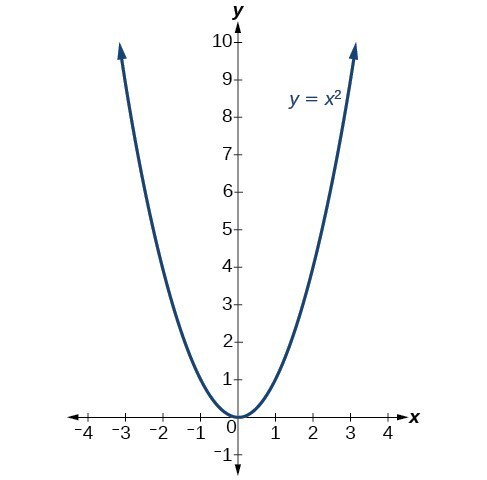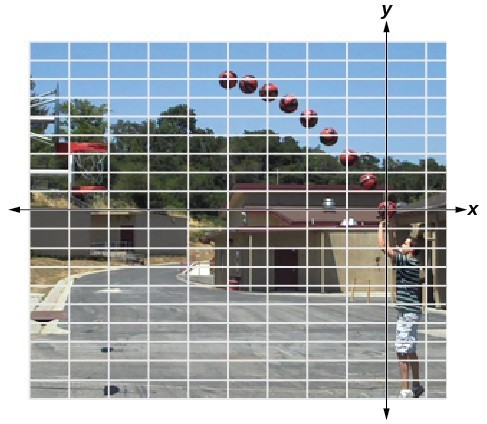### Learning Outcomes

• Graph vertical and horizontal shifts of quadratic functions
• Graph vertical compressions and stretches of quadratic functions
• Write the equation of a transformed quadratic function using the vertex form
• Identify the vertex and axis of symmetry for a given quadratic function in vertex form

The standard form of a quadratic function presents the function in the form

$f\left(x\right)=a{\left(x-h\right)}^{2}+k$

where $\left(h,\text{ }k\right)$ is the vertex. Because the vertex appears in the standard form of the quadratic function, this form is also known as the vertex form of a quadratic function.

The standard form is useful for determining how the graph is transformed from the graph of $y={x}^{2}$. The figure below is the graph of this basic function.## Shift Up and Down by Changing the Value of $k$

You can represent a vertical (up, down) shift of the graph of $f(x)=x^2$ by adding or subtracting a constant, $k$.

$f(x)=x^2 + k$

If $k>0$, the graph shifts upward, whereas if $k<0$, the graph shifts downward.

### Example

Determine the equation for the graph of $f(x)=x^2$ that has been shifted up 4 units. Also, determine the equation for the graph of $f(x)=x^2$ that has been shifted down 4 units.

The equation for the graph of $f(x)=x^2$ that has been shifted up 4 units is

$f(x)=x^2+4$

The equation for the graph of $f(x)=x^2$ that has been shifted down 4 units is

$f(x)=x^2-4$

## Shift left and right by changing the value of $h$

You can represent a horizontal (left, right) shift of the graph of $f(x)=x^2$ by adding or subtracting a constant, $h$, to the variable $x$, before squaring.

$f(x)=(x-h)^2$

If $h>0$, the graph shifts toward the right and if $h<0$, the graph shifts to the left.

### Example

Determine the equation for the graph of $f(x)=x^2$ that has been shifted right 2 units. Also, determine the equation for the graph of $f(x)=x^2$ that has been shifted left 2 units.

The equation for the graph of $f(x)=x^2$ that has been shifted right 2 units is

$f(x)=(x-2)^2$

The equation for the graph of $f(x)=^2$ that has been shifted left 2 units is

$f(x)=(x+2)^2$

## Stretch or compress by changing the value of $a$.

You can represent a stretch or compression (narrowing, widening) of the graph of $f(x)=x^2$ by multiplying the squared variable by a constant, $a$.

$f(x)=ax^2$

The magnitude of $a$ indicates the stretch of the graph. If $|a|>1$, the point associated with a particular $x$-value shifts farther from the $x$–axis, so the graph appears to become narrower, and there is a vertical stretch. But if $|a|<1$, the point associated with a particular $x$-value shifts closer to the $x$–axis, so the graph appears to become wider, but in fact there is a vertical compression.

### Example

Determine the equation for the graph of $f(x)=x^2$ that has been compressed vertically by a factor of $\frac{1}{2}$. Also, determine the equation for the graph of $f(x)=x^2$ that has been vertically stretched by a factor of 3.

The equation for the graph of $f(x)=x^2$ that has been compressed vertically by a factor of $\frac{1}{2}$ is

$f(x)=\frac{1}{2}x^2$

The equation for the graph of $f(x)=x^2$ that has been vertically stretched by a factor of 3 is

$f(x)=3x^2$

The standard form and the general form are equivalent methods of describing the same function. We can see this by expanding out the general form and setting it equal to the standard form.

This is the $x$ coordinate of the vertex and $x=-\dfrac{b}{2a}$ is the axis of symmetry we defined earlier. Setting the constant terms equal gives us:

In practice, though, it is usually easier to remember that $h$ is the output value of the function when the input is $h$, so $f\left(h\right)=f\left(-\dfrac{b}{2a}\right)=k$.

### Try It

A coordinate grid has been superimposed over the quadratic path of a basketball in the picture below. Find an equation for the path of the ball. Does the shooter make the basket?(credit: modification of work by Dan Meyer)

The path passes through the origin and has vertex at $\left(-4,\text{ }7\right)$, so $\left(h\right)x=-\frac{7}{16}{\left(x+4\right)}^{2}+7$. To make the shot, $h\left(-7.5\right)$ would need to be about 4 but $h\left(-7.5\right)\approx 1.64$; he doesn’t make it.

You are watching: Transformations of Quadratic Functions. Info created by GBee English Center selection and synthesis along with other related topics.